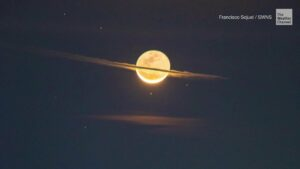# Week 3 – OOP(s) Moon

Concept & Inspiration:

This code creates a graphical representation of a moon with an orbit. The code defines a class called “Moon” that creates an instance of a moon with given x, y coordinates, width, and height. The class has a method called “draw” which draws the moon on the canvas. The code also defines a class called “Square” which creates a square with given x, y coordinates, size and color. This class also has a method called “draw” which draws the square on the canvas.The code uses an array “squares” to store instances of the square class and uses a loop to draw multiple squares in an orbit around the moon. The squares are positioned using the cosine and sine functions to make them move in a circular motion.The code also allows for the creation of stars by making an array “stars” and using a loop to draw 50 small circles on the canvas. The stars can be displayed by clicking on the canvas.The function “setup” initializes the canvas, sets the frame rate and creates the instances of the moon and squares.The function “draw” displays the moon and squares on the canvas. The function “mouseClicked” changes the display of stars when the mouse is clicked on the canvas.

This code captures the essence of a celestial body inspired by the rings of Saturn. The inspiration for this code came from an image of the moon surrounded by clouds, which gave the illusion of it having its own set of rings. With a creative and imaginative approach, the goal was to bring to life a moon with an animated orbit. The picture of the image is shown below.Code:

```let star = false; // when there are no stars
let stars = []; //making an array for storing stars
class moon{ // class for making a moon
constructor(xm, ym, wm, hm,cm) {
this.xm = xm; // x coordinate of moon
this.ym = ym; // y coordinate of moon
this.wm = wm; // width of moon
this.hm = hm; //height of moon
}

draw() {
// drawing the moon itself with craters
fill("lightgrey");
ellipse(this.xm, this.ym, this.wm, this.hm);
noStroke();
ellipse(this.xm + 20, this.ym-10, this.wm/5, this.hm/4);
ellipse(this.xm -15, this.ym+10, this.wm/5, this.hm/4);
ellipse(this.xm -10 , this.ym-20, this.wm/5, this.hm/5);
ellipse(this.xm +15 , this.ym+12, this.wm/5, this.hm/5);
}

}
class Square { // making a class for squares for the orbit
constructor(x, y, size, colors) {
this.x = x; // x coordinate
this.y = y; // y coordinate
this.size = size; // size of squares
this.colors = colors; //color of square
}

draw() { //drawing a square
strokeWeight(1);
fill(this.colors);
rect(this.x, this.y, this.size, this.size);
}
}

let squares = []; //array of squares to store them
let angle = 0;
let angleStep = 5;
let xoffset = 0;
let yoffset = 0;

let moon_obj = new moon(200,200,75,75,100,200); //making a moon with the help of a moon class

function setup() {
createCanvas(400, 400);
frameRate(50);
//making the orbit with squares which are very tiny and using sin and cos functions to make them move in a particular motion
for (let i = 0; i < 1000; i++) {
let x = width/2 + radius * cos(angle);
let y = height/2 + radius * sin(angle);
let size = 2;
let colors = (random(200), random(200), random(255));
squares.push(new Square(x, y, size, colors));

angle += angleStep;

}

// to make stars which appear upon clicking on the canvas
for (let i = 0; i < 50; i++) {
stars[i] = {
x: random(0, width),
y: random(0, height)
};
}
}

function draw() { // displaying my creative idea of a moon with an orbit
if(star==false)
{
background(0);

for (let i = 0; i < squares.length; i++) {
squares[i].x = squares[i].x + xoffset + sin( frameCount*0.01+i*0.1);
squares[i].y = squares[i].y + yoffset + cos(frameCount * 0.05 + i * 0.1);
squares[i].draw();
}
moon_obj.draw();
}

else // if clicked displaying moon as imagined by many
{
background(0);
moon_obj.draw();
fill("white");
for (let i = 0; i < 50; i++) {
ellipse(stars[i].x, stars[i].y, 1, 1);
}
}

}

function mouseClicked() {

if(star==false)
{
star =true;
}
else
star =false;
}```

Reflection / Future Improvements:

The project was really fun to work on as I learned how to deal with classes and arrays. Making the entire thing function was hard and took a lot of time to understand the concept better. The code creates a beautiful representation of a moon with rings similar to that of Saturn. The code uses classes for the moon and the rings to make it organized and reusable. The rings are created using a series of small squares that are animated in an orbital pattern around the moon. The use of sin and cos functions adds a touch of realism to the motion of the rings. Additionally, the user can toggle between a starry night sky or a moon with rings with a single mouse click.

However, there are a few areas for improvement in this code. First, the color scheme of the moon and the rings could be made more dynamic by using random colors or a gradient effect. Second, the stars in the starry night sky could be made better to make it look more realistic. Lastly, the motion of the rings could be made more dynamic by adding an acceleration or deceleration effect. These improvements would enhance the overall look and feel of the code and make it even more visually appealing.